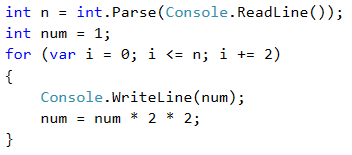# Example: Even Powers of 2

Print the even powers of 2 to 2^n: 2^0, 2^2, 2^4, 2^8, …, 2^n. For example, if n = 10, the result will be: 1, 4, 16, 64, 256, 1024.

## Video: Even Powers of 2

Watch this video lesson to learn how to print the even powers of 2 using a for loop: https://youtu.be/H8t4HGn_Ap4.

## Hints and Guidelines

Here is how we can solve the problem using a for-loop with a step:

• We create a num variable for the current number to which we assign an initial value of 1.
• For a step of the loop, we set a value of 2.
• In the body of the loop: we print the value of the current number and increase the current number num 4 times (according to the problem's description).